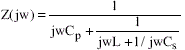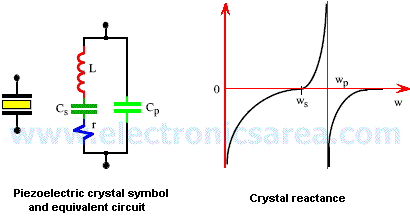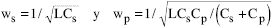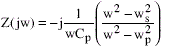# The Crystal Oscillators (Piezoelectric Oscillator)

A quartz crystal has a property called: piezoelectric effect. This effect causes, by applying a mechanical pressure on the surface of the crystal, that a voltage is developed on the opposite sides. In a similar way, a voltage applied on the faces of the crystal produces a mechanical distortion on their surface.

An AC voltage causes mechanical vibration whose natural frequency is very stable and depends on the nature and carved crystal.

## Crystal Oscillator equivalent circuit

The equivalent circuit model of a quartz crystal (look at the picture below) is characterized by a very large inductance L (several Henrys), a very small capacitance Cs (<0.5 pF) in series, and a series resistance r (a few hundred ohms), and a parallel capacity Cp (a few pF) which represents the electrostatic capacity between the two sides of the crystal.

The quality factor Q is high (> 20000). If “r” is neglected, the crystal impedance Z (jw) is given by:The equation above indicates that the crystal has two resonant frequencies: a series resonance “ws” and a parallel resonance “wp” given by the following equations:You may also like: Resonance in a RLC Circuits

The last equation can be rewritten in the form:where wp > ws since Cp > Cp.

A graphical representation of the reactance (impedance) Z (jw) is shown on picture above.

•
•
•
•
•
•
•
•
•
•
•
•
•
•
•
•